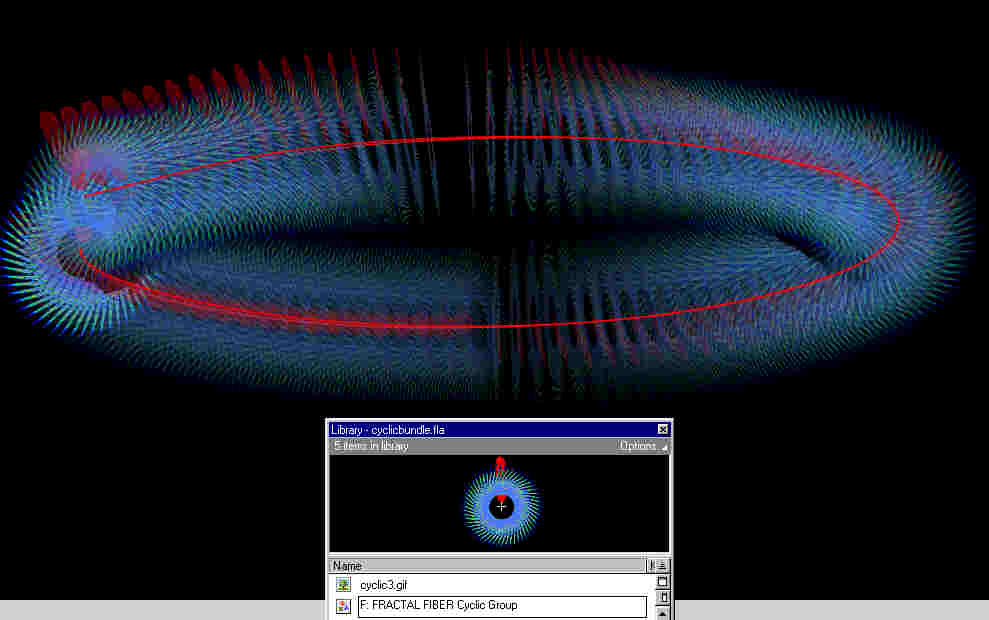Constructing a Fiber Bundle with Fractal Fibers
( an imperfect model with no immediate application )
Constructed here is a fiber bundle over a Base Space comprised of a circle S1 with Fiber given by a fractal with Zn cyclic symmetry group ( n = degree of cyclical symmetry ) and produced by an Iterative Function System, IFS.  The Total Space E is comprised of the fractal parametrically winding or twisting about it's local center and following the global orbit line around a non-local axis until it matches back to the Fractal Fiber's starting position.

The angle of local Twistcan be in integral multiples of N*(360/n), where { N = 0, 1, 2 ...} and { n = degree of cyclic symmetry}. A Fiber Bundle is said to have a TwistT which can determine the orientability of the Total Space. The Twist Group invariants, soliton, abelian, non-abelian, trivial, non-trivial, Gauss-Bonnet, symplectic, Gauge Group,

Fiber Bundles are spaces which are locally Products but not necessarily globally.  A discrete fiber space produces a fibration that is a covering space, like a helix covering a circle. A vector fiber space creates a vector bundle and is classified either algebraically by characteristic classes which are elements of homology groups OR geometrically by maps to classifying spaces. Also, a loop space produces the space of loops in a given space.

These invariants are called characteristic classes and are viewed formally as elements in the cohomology groups of the manifold, the duals of the homology groups. Characteristic classes can also be defined purely topologically by investigating the global motion of the tangent space as the manifold is traversed. They measure to some extent the failure of the manifold to possess a globally defined notion of parallelism: for example, a global parallelism exists on the torus in which Euler-Poincaré characteristic = 0, but not on the two-dimensional sphere in which Euler-Poincaré characteristic = 2.

(... the Euler-Poincaré characteristic number is related to the Euler graph number for the graph that is related to the surface ? ...)

Fibers are objects in some Category. All fibers must be isomorphic, homeomorphic, or self-similar. Every Category is built with a notion of isomorphism.  Vector Spaces, Groups, Topological Spaces, Manifolds, G-sets, and Fractals.  Two Fibers are homeomorphic if one Fiber can be deformed into another by a one-to-one mapping and a continuous function. Homeomorphism is too weak to preserve the fractal nature of objects.

For example, the Koch curve is actually homeomorphic to the plain old non-fractal line segment of length 1.

People usually talk about self-similar fractals. A similarity is stronger than a homeomorphism. A one-to-one and onto mapping is called a similarity if there is a constant c (called the similarity factor) such that for any
two points x1 and x2 in X their images y1 and y2 in Y have the property that the distance from y1 to y2 is c times the distance from x1 to x2.

A fractal is called self-similar if it it can bew divided into finitely many pieces :

X = X1 union X2 union ....

such that there is a similarity f1 from X onto X1, a similarity f2 from X onto X2 and so on.

In that sense the Koch curve is self-similar because it can be divided into four pieces, each of which is 1/3 the size of the original Koch curve.

A Fiber Bundle can be a Fibration, but not necessarily everytime.

( ... something about the parallel fibres .... in the special case of the affine space where the connection ..., parallelism of Aji means the constancy of all the components.  Properties of the connections imply certain types of manifolds falling in certain types of characteristic classes ... parallel transport ...

Connection A <> Curvature dA <> Torsion A ^ dA <> Hypervolume dA ^ dA ...
Gauge Theory <> Connection Manifold <> Choice of A - Gauge Freedom)
Geodesics, Foliations with Leaves, (non) Anosov Flow > Ergodicity > Topo Entropy, diffeomorphism, orbits, orbitfolds, orientifolds, Laminations

Each Fiber F in the Total Space E projects to exactly one point in the Base Space B.  The Product of a Fiber F and a local Neighborhood U of the Base Space B creates the Total Space E.   The Product can be denoted as
U x F ->E or  B x F->E.

The inverse image of a point in the Base Space B is the Fiber F .

A Fiber Bundle with a Fractal as a Fiber F is a Map f : E ->where E is called the Total Space of the fiber bundle and B the Base Space of the fiber bundle.  Showing that for every point in the Base Space ( b in B ) has a Neighborhood  U so that   f1(U) is Homeomorphic to U x F by some continuous function and one-to-one mapping.

Moebius Twisted Ring with Fractal Cross-Section
Scroll Down for Image ( sounds effects will be removed in the future ) :
If you cannot see the above flash object, goto MacroMedia's homepage to download a free Shockwave player...or see the below picture ...Troy Christensen
Email